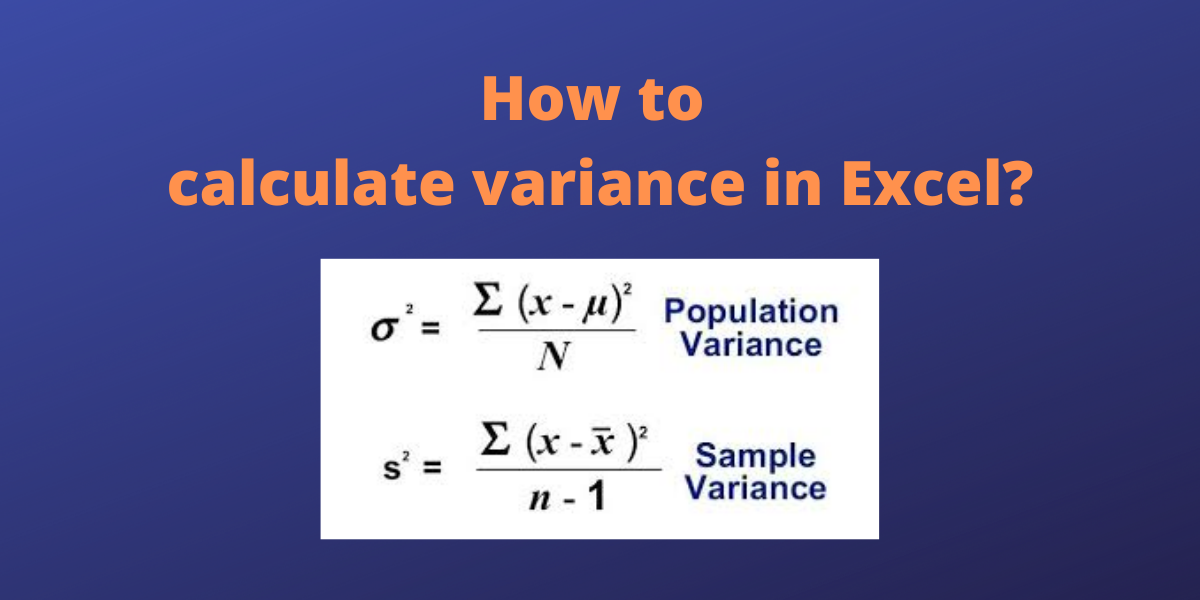# How to Calculate Variance in Excel?Variance is a statistical measurement that measures the spread between data points in a data set. It is defined mathematically as the average of the squared deviations from the mean. The formula for sample variance and populations variance is

where x is a data point, x bar is the mean of the data points in the data set, and n is the number of data points in the data set.

We can calculate variance in Excel both using the mathematical formula and built-in functions.

## Using the Variance Mathematical Formula in Excel

Let us consider a sample data set containing integer values for which variance is sought.

First, we need to calculate the mean of the numbers as the mean is a parameter in the formula for variance. To calculate the mean in Excel, we use an in-built AVERAGE function. The syntax of the AVERAGE function is =AVERAGE(start cell reference:end cell reference). Follow these steps to calculate the mean in Excel:

• Select the cell where you want to display the mean.
• Type the formula =AVERAGE( and select the data range containing the values for which mean value is sought.
• Finish the formula with ). Here, the numbers are present in cells from A2 to A11, so we type =AVERAGE(A2:A11) in the formula bar.
• Press the Enter key to calculate and display the result.

Now that we have calculated the mean, we need to calculate the deviation of individual data points from the mean. The deviations are calculated in the column right next to the data points. Follow these steps to calculate the deviations:

• Select the cell right next to the first data point. In this example, this cell is B2.
• To get the difference, type =A2-\$B\$13, the \$ sign makes the value constant.
• Press the Enter key to display the result.
• Simply copy the formula for the entire list to calculate the deviation for every data point by dragging down the fill handle.

Next, we need to calculate the squares of the deviations. This can be calculated using the caret operator ^. Follow these steps to find the squares of the deviations in the column next to it:

• Select the cell right next to the first deviation. In this example, this cell is C2.
• To get the square, type =B2^2 in the formula bar.
• Press the Enter key to display the result.
• Simply copy the formula for the entire list to calculate the square of deviation for every data point by dragging down the fill handle.

We now need to calculate the sum of the square of deviations. We can do this by using an inbuilt function SUM in Excel. Follow these steps to calculate the sum of all deviations:

• Go to the cell where you want to display the sum.
• Type the formula =SUM( and select the data range containing the values for which summation value is sought.
• Finish the formula with ). Here, the deviations are present in cells from C2 to C11, so we type =SUM(C2:C11) in the formula bar.
• Press the Enter key to display the calculated sum.

Sample variance is the sum of squares of deviations divided by (n -1), where n is the number of data points in the sample. Follow these steps to calculate the variance of the sample:

• Go to the cell where you want to display the variance.
• Type =B14/(10-1), where B14 contains the sum of squares of deviations.
• Press the Enter key to display the calculated result.

Population variance is the sum of squares of deviations divided by n, where n is the number of data points in the sample. Follow these steps to calculate the variance of the population:

• Go to the cell where you want to display the variance.
• Type =B14/10, where B14 contains the sum of squares of deviations.
• Press the Enter key to display the calculated result.

## Using the VAR.S and VAR.P function

In Excel, an inbuilt function VAR.S calculates variance based on a sample. Syntax: VAR.S(n), where n is the list of numbers or reference to the cell ranges containing the numbers.

• Select the cell where you want to display the sample variance.
• Type the formula =VAR.S( and select the data range containing the values for which sample variance is sought.
• Finish the formula with ). Here, the numbers are present in cells from A2 to A11, so we type =VAR.S(A2:A11) in the formula bar.
• Press the Enter key to display the calculated result.

Note: The VAR.S function ignores logical values and text in the sample.

In Excel, an inbuilt function VAR.P calculates variance based on the entire population. Syntax: VAR.P(n), where n is the list of numbers or reference to the cell ranges containing the numbers.

• Select the cell where you want to display the population variance.
• Type the formula =VAR.P( and select the data range containing the values for which population variance is sought.
• Finish the formula with ). Here, the numbers are present in cells from A2 to A11, so we type =VAR.P(A2:A11) in the formula bar.
• Press the enter key to display the calculated result.

Note: The VAR.P function ignores logical values and text in the sample.

## Conclusion

In this tutorial, we learned how to calculate sample and population variance both by using mathematical formulas and inbuilt functions VAR.S and VAR.P in Excel.